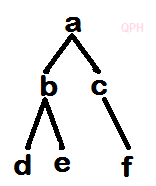# DBATU Dec 2019 Data structure Question Paper

QuestionPapersHub

###### Stay connected :BABASAHEB AMBEDKAR TECHNOLOGICAL UNIVERSITY, LONERE.

End Semester Examination – May 2019

Branch: B. Tech ( Computer Engineering)-Sem-3

Subject (Subject Code): Data Structures (BTCOC303)

Date: 14/12/2019

Time: 3 Hrs.

Marks: 60

Instructions to the Students :

1. Solve ANY FIVE questions out of the.
2. The level of question/expected answer as per OBE or the Course Outcome on which the question is based is mentioned in front of the.
3. Use of non-programmable scientific calculators is allowed.
4. Assume suitable data wherever necessary & mention it clearly.

Q.1. Attempt any 3(4×3=12).

a) Why to study data structures? What are the major data structures used in the RDBMS, Network & Hierarchical data model.

b) Consider the following specification of a graph G= (V, E)

V= {1, 2, 3, 4}

E= {(1, 2), (1, 3),(3, 3),(3, 4),(4, 1)}

i. Draw an undirected graph.

ii. Represent graph G using adjacency matrix.

c) Suppose the numbers : 50, 15, 62, 5, 20, 58, 91, 3, 8, 37, 60, 24 are inserted in order into an initially empty binary search tree. What is preorder, inorder & postorder transversal sequence of the tree?

d) What is garbage collection? Who will run garbage collection program? When it will be run?

Q.2. Solve all the following  questions(4×3=12).

a) What is sparse matrix? Convert the following sparse matrix.

1 0 0 0

0 -2 11 0

0 0 0 0

0 6 0 5

b) Suppose multidimensional arrays A & B are declared using

A (-1:3) & B (1:5, -3.1).

Find the length of each dimension &  the number of elements in A & B.

p(x)=2x8-5x7+3x2+4 .

d) What is hash data structure?The keys: 32, 18, 23, 2, 3, 44, 5 and 15 are inserted into an initially empty hash table of length 10 with hash function H(Key)= key mod 10 & linear probing is used to resolve collision. What is hash table content after every key insertion?

Q.3. Solve any 3 of the following questions(3×4=12).

a) Give an algorithm to complement binary search with its advantages & disadvantages.

b) Explain the concept of skip list with an example. Give its advantages & disadvantages.

c) Sort the following list using radix sort. Show all the passes neatly:

3 45 7 18 9 4 89 103 11 21

d) Suppose we are sorting an array of eight integers using quick-sort, and we have just finished the first partitioning with the array looking like this:

2, 5, 1, 7, 9, 12, 21, 30

What was the pivot element in the first partition? Also complete the rest of the partitions so that all numbers will be in the ascending order.

Q.4. Solve any 2 of the following (2×6=12).

a) Write an algortihm to insert a new node at the begining of the singly linked list.

b) What is singly circular linked list? Write an algorithm to transverse the list & also enlist different operations performed on it.

c) Write a short note on dynamic storage management. Explain how it is done in C.

Q.5. Solve any 2 (2×6=12).

a) Consider the stack where N=6 memory cells allocated. Suppose initially stack contains A, D, E, F, G (Top of stack). Then the following operatins called in order. Show the stack top & any other situation raised while doing each of the operations.

i) Push (Stack, K)

ii) Pop(Stack, Item)

iii) Push(Stack, L )

iv) Push(Stack, S)

v) Pop(Stack, Item)

vi) Push(Stack, T)

b) What is queue? Write an algorithm to implement insert item into queue using singly linked list.

c) write an algorithm to evaluate postfix expression using stack & execute your algorithm with postfix expression 10, 5, 6, *, +, 8, /.

Show intermediate stack content after each operation.

Q.6. Solve all of the following(4×3=12).

a) Give the characteristics of good algorithm. Also explain how do e analyze the algorithm.

b) Store elements of the given below binary tree using

i) One dimensional array